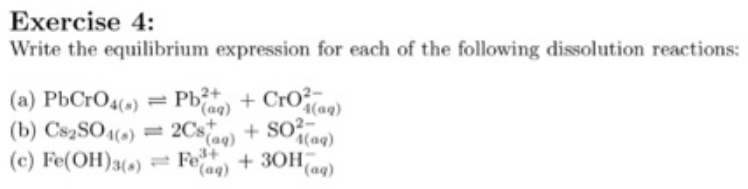# Problem: Write the equilibrium expression for each of the following dissolution reactions: (a) PbCrO4 (s) ⇌ Pb2+ (aq) + CrO42- (aq)(b) Cs2SO4 (s) ⇌ 2Cs+ (aq) + SO42-(aq) (c) Fe(OH)3 (s) ⇌ Fe3+ (aq) + 3OH- (aq)

###### FREE Expert Solution
92% (424 ratings)###### Problem Details

Write the equilibrium expression for each of the following dissolution reactions:

(a) PbCrO4 (s) ⇌ Pb2+ (aq) + CrO42- (aq)

(b) Cs2SO4 (s) ⇌ 2Cs(aq) + SO42-(aq)

(c) Fe(OH)3 (s) ⇌ Fe3+ (aq) + 3OH- (aq)